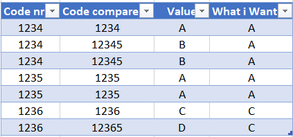cancel
Showing results for
Did you mean:Frequent Visitor

## xlookup problem

Hello,

I'm having problems getting the same results in power query or powerbi as im getting in excel.

In the original table I have 3 columns. I need to create a new column "What i want".

As you can see, the column "Value" depends on the column "Code Compare" but what I need is a new column that gets the "Value" according to the column "Code nr".In Excel I'm using the following code:

xlookup([@[Code nr]]; [Code compare]; [Value]; "";0)

I tried to use the lookupvalue function in dax but with no success.

Thank you.

2 ACCEPTED SOLUTIONSSuper User

Hello - this is how you can get the result expected with Power Query:

``Table.AddColumn(#"Changed Type", "Custom", each Table.SelectRows(#"Changed Type", (x)=> x[Code compare]=[Code nr] ){0}[Value])``Super User

Thanks for posting the script.  You are getting additional rows when you expand the tables in the last step, is because the previous step, in which the new column is added, is returning a table in each row, and that table has more than one row. When you expand the column of tables, each row in the original table is expanded by the number of rows that are in the table in each F_origin.  You will need to either filter the rows in each F_origin prior to expanding, like this:

``````Table.TransformColumns (
{
{
"F_origin",
(t) => Table.SelectRows ( t, each t[Column1] = "Your critieria here" )
}
}
)``````

Or drill down further when you add F_origin as a new column, like this:

``Table.AddColumn(#"Preenchido para Baixo", "F_origin", each Table.AddColumn(#"Preenchido para Baixo", "Custom", each Table.SelectRows(#"Preenchido para Baixo", (x)=> x[Ord. Fabrico]=[#"Ord. Fabrico (Origem)"] ){0}[Formato][ColumnName]{RowIndexBase0}))``

7 REPLIES 7Super User

Hello - this is how you can get the result expected with Power Query:

``Table.AddColumn(#"Changed Type", "Custom", each Table.SelectRows(#"Changed Type", (x)=> x[Code compare]=[Code nr] ){0}[Value])``Frequent Visitor

Hello @jennratten , thank you for replying.

I got the result using your solution but now powerquery is duplicating the row values.Super User

Can you please post your script?  That will help identify why the rows are being duplicated.Frequent Visitor

Hi @jennratten ,

I created two pages, one with a table named "what i need" and the other with "what i dont need".

The second one is the powerquery attempt.

Thank youSuper User

Hello - I am unable to download the file you attached.  Can you instead post your script here?  For your query table, click Advanced Editor from the ribbon in the query editor, copy the text, click the script button in the response message here and paste the script.  Thanks!Frequent Visitor

Hello @jennratten ,Super User

Thanks for posting the script.  You are getting additional rows when you expand the tables in the last step, is because the previous step, in which the new column is added, is returning a table in each row, and that table has more than one row. When you expand the column of tables, each row in the original table is expanded by the number of rows that are in the table in each F_origin.  You will need to either filter the rows in each F_origin prior to expanding, like this:

``````Table.TransformColumns (
{
{
"F_origin",
(t) => Table.SelectRows ( t, each t[Column1] = "Your critieria here" )
}
}
)``````

Or drill down further when you add F_origin as a new column, like this:

``Table.AddColumn(#"Preenchido para Baixo", "F_origin", each Table.AddColumn(#"Preenchido para Baixo", "Custom", each Table.SelectRows(#"Preenchido para Baixo", (x)=> x[Ord. Fabrico]=[#"Ord. Fabrico (Origem)"] ){0}[Formato][ColumnName]{RowIndexBase0}))``